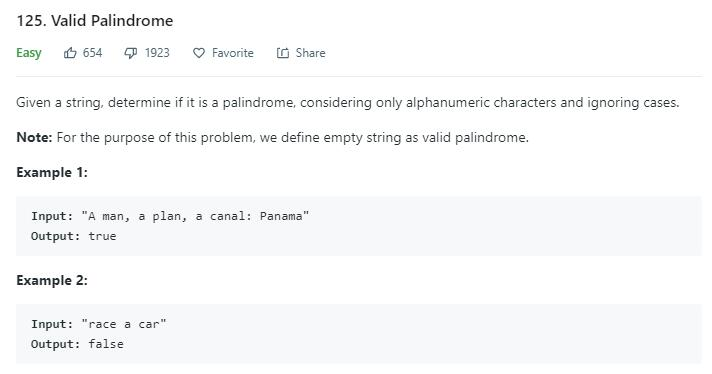# 题目描述（简单难度）# 解法一

public boolean isPalindrome(String s) {
int len = s.length();
s = s.toLowerCase(); //统一转为小写
int i = 0, j = len - 1;
while (i < j) {
//跳过非法字符
while (!isAlphanumeric(s.charAt(i))) {
i++;
//匹配 "   " 这样的空白字符串防止越界
if (i == len) {
return true;
}
}
while (!isAlphanumeric(s.charAt(j))) {
j--;
}
if (s.charAt(i) != s.charAt(j)) {
return false;
}
i++;
j--;
}
return true;
}

private boolean isAlphanumeric(char c) {
if ('a' <= c && c <= 'z' || 'A' <= c && c <= 'Z' || '0' <= c && c <= '9') {
return true;
}
return false;
}


# 解法二

private static final char[] charMap = new char;

static {
// 映射 '0' 到 '9'
for (int i = 0; i < 10; i++) {
charMap[i + '0'] = (char) (1 + i); // numeric
}
// 映射 'a' 到 'z' 和 映射 'A' 到 'Z'
for (int i = 0; i < 26; i++) {
charMap[i + 'a'] = charMap[i + 'A'] = (char) (11 + i);
}
}

public boolean isPalindrome(String s) {
char[] pChars = s.toCharArray();
int start = 0, end = pChars.length - 1;
char cS, cE;
while (start < end) {
// 得到映射后的数字
cS = charMap[pChars[start]];
cE = charMap[pChars[end]];
if (cS != 0 && cE != 0) {
if (cS != cE)
return false;
start++;
end--;
} else {
if (cS == 0)
start++;
if (cE == 0)
end--;
}
}
return true;
}